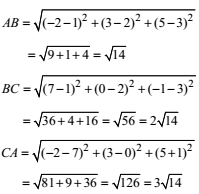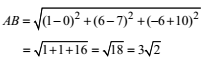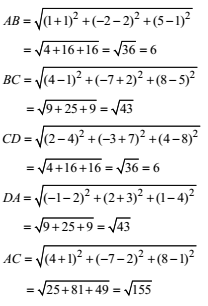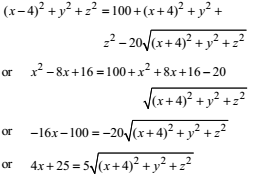## NCERT Solutions for Class 11 Maths Chapter 12 Introduction to Three Dimensional Geometry Exercise 12.2

NCERT Solutions of Chapter 12 Introduction to Three Dimensional Geometry Exercise 12.2 is very helpful if you want to crack examination with good marks. The subject matters experts of Studyrankers have prepared best accurate and detailed NCERT Solutions for Class 11 Maths which will be useful for you in understanding the concepts embedded in a question.1. Find the distance between the following pairs of points:
(i) (2, 3, 5), (4, 3, 1)
(ii) (–3, 7, 2), (2, 4, –1)
(iii) (–1, 3, –4), (1, –3, 4)
(iv) (2, –1, 3), (–2, 1, 3)

(i) The given points are (2, 3, 5) and (4, 3, 1).
Required distance
= √((4 – 2)2 + (3 – 3)2 + (1– 5)2)
= √(4 + 0 + 16) = √20 = 2√5
(ii) The given points are (–3, 7, 2) and (2, 4, –1).
Required distance
= √( (2 + 3)2 + (4 – 7)2 + (–1– 2)2)
= √(25 + 9 + 9) = √43
(iii) The given points are (–1, 3, – 4) and (1, –3, 4).
Required distance
= √( (1 + 1)2 + (–3 – 3)2 + (4 + 4)2)
= √(4 + 36 + 64) = √104 = 2√26
(iv) The given points are (2, –1, 3) and (–2, 1, 3).
Required distance
= √((2 + 2)2 + (-1 - 1)2 + (3 - 3)2)
= √(16 + 4 + 0) = √20 = 2√5

2. Show that the points (–2, 3, 5), (1, 2, 3) and (7, 0, –1) are collinear.

Let the given points be A(–2, 3, 5), B(1, 2, 3) and C(7, 0, –1).Thus AB + BC = AC
Hence, the points A, B, C are collinear.

3. Verify the following
(i) (0, 7, – 10), (1, 6, – 6) and (4, 9, –6) are the verticles of an isosceles triangle.
(ii) (0, 7, 10), (–1, 6, 6) and (– 4, 9, 6) are the vertices of a right angled triangle.
(iii) Verify the given coordinates (–1, 2, 1), (1, –2, 5) (4, –7, 8) and (2, –3, 4) are the vertices of a parallelogram.

(i) Let A(0, 7, – 10), B(1, 6, –6) and C(4, 9, –6) are the given vertices.
NowandThus, AB = BC. Hence A, B and C are the vertices of an isosceles triangle.

(ii) Let the vertices be A(0, 7, 10), B(–1, 6, 6) and C(–4, 9, 6).Hence A, B and C are the vertices of a rightangled triangle.

(iii) The given points are A(–1, 2, 1), B(1, –2, 5), C(4, –7, 8) and D(2, –3, 4), then by distance formulaNow, since AB = CD and BC = DA i.e., opposite sides are equal and AC ≠ BD  i.e. the diagonals are not equal. So, points are the vertices of parallelogram.

4. Find the equation of the set of points P, which are equidistant from (1, 2, 3) and (3, 2, –1).

Let A(1, 2, 3) and B(3, 2, – 1) be the given points and the coordinates of points P be (x, y, z) We are given AP = BP or AP2 = BP25. Find the equation of the set of points P, the sum of whose distances from A(4, 0, 0) and B(– 4, 0, 0) is equal to 10.